Capital Budgeting | IFT World
IFT Notes for Level I CFA® Program
IFT Notes for Level I CFA® Program

# Part 2

## 3. The Capital Allocation Process

Capital allocation is the process used by an issuer’s management to make capital investment decisions.

Steps in Capital Allocation Process

The steps in the capital allocation process are as follows:

Step 1 – Idea generation: Most important step in the process. Investment ideas can come from anywhere within the organization, or outside (customers, vendors, etc.). What projects can add value to the company in the long term?

Step 2 – Investment analysis: Gathering information to forecast cash flows for each project and then computing the project’s profitability. Output of this step: A list of profitable projects.

Step 3 – Capital allocation planning: Do the profitable projects fit in with the company’s long-term strategy? Is the timing appropriate? Some projects may be profitable in isolation but not so much when considered along with the other projects. Scheduling and prioritizing of projects are important.

Step 4 – Monitoring and post-audit: Post-audit helps in assessing how effective the capital budgeting process was. How do the actual revenues, expenses, and cash flows compare against the predictions? Post-auditing is useful in three ways:

• If the predictions were optimistic or too conservative, then it becomes evident here.
• Helps improve business operations. Puts the focus on out-of-line sales and costs.
• Helps in identifying profitable areas for fresh investments in the future, or scale down in non-profitable ones.

Capital Allocation Principles

The following are the six key principles of capital allocation:

1. Decisions are based on cash flows: Decisions are not based on an accounting/accrual basis (net income or operating income) which subtract non-cash charges such as depreciation.
1. Measure incremental cash flows: What incremental cash flows occur with the investment as compared to without it?
2. Timing of cash flows is crucial: Due to the time value of money, cash flows received earlier are more valuable than cash flows received later.
1. Cash flows are analyzed on an after-tax basis: Shareholder value increases only on the cash that they have earned. Hence, any tax expenses must be deducted from the cash flows.
1. Cash flows are not accounting net income or operating income: Cash flows should not reflect non-cash charges. Economic income (cash inflows plus change in company’s market value),unlike accounting income, does not reflect non-cash charges.)
2. Financial costs are ignored: Financial costs are already included in the cost of capital (discount rates) used to discount cash flows to arrive at the present value. Hence, to avoid double counting, they must not be deducted from the cash flows.

Other important points to consider:

• Exclude sunk costs: A sunk cost is one that has already been incurred and cannot be changed. For example, already incurred costs like preliminary consulting fees should not be included in the analysis.
• Use incremental cash flows: Cash flow with the decision minus the cash flow without the decision.
• Include externalities: Both positive/negative externalities should be considered in the analysis. Cannibalization is an example of a negative externality. For example, negative impact of a new diet soda product launch on the sales of existing soda products.
• Conventional cash flow pattern vs. nonconventional cash flow pattern: A project has conventional cash flows if the sign of cash flows changes only once during the life of the project, while an unconventional cash flow project has more than one sign change.

Independent projects vs. mutually exclusive projects: Independent projects are unrelated projects that can be analyzed separately, while mutually exclusive projects compete with each other. Two independent projects can both be executed if they individually meet the criteria. If two projects are mutually exclusive, then either of the two can be undertaken, not both.

Project sequencing: Certain projects are linked through time, i.e., completion of one project creates an opportunity to invest in another project later based on its profitability.

## 4. Investment Decision Criteria

### Net Present Value

Net present value is the present value of the future after tax cash flows minus the investment outlay.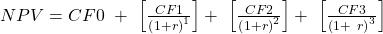Decision rule:

For independent projects:

If NPV > 0, accept.

If NPV < 0, reject.

For mutually exclusive projects:

Accept the project with higher and positive NPV.

Example

Compute NPV for projects A and B given the following data:

 Cost of capital = 10% Expected Net after Tax cash flows Year Project A (in $) Project B (in$) 0 -1,000 -1,000 1 500 100 2 400 300 3 300 400 4 100 600

Solution:

Project A:

NPV =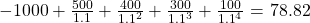On the exam, you can save time by using the calculator to solve for NPV instead of using the above formula. The key strokes are given below:

 Key strokes Display [CF][2nd][CLR WORK] CF0 = 0 1000 [+|-] [ENTER] CF0 = -1000 [↓] 500 [ENTER] C01 = 500 [↓] F01 = 1 [↓] 400 [ENTER] C02 = 400 [↓] F02 = 1 [↓] 300 [ENTER] C03 = 300 [↓] F03 = 1 [↓] 100 [ENTER] C04 = 100 [↓] F04 = 1 [NPV] 10 [ENTER] I = 10 [↓] CPT NPV = 78.82

Project B:

NPV =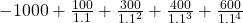=49.18

Microsoft Excel functions can also be used to solve for the NPV. The two functions available are:

• NPV or =NPV(rate, values) and
• XNPV or =XNPV(rate, values, dates),

where “rate” is the discount rate, “values” are the cash flows, and “dates” are the dates of each of the cash flows.

### Internal Rate of Return

IRR is the discount rate that makes the present value of future cash flows equal to the investment outlay. We can also say that IRR is the discount rate which makes NPV equal to 0.

Decision rule:

For independent projects:

If IRR > required rate of return (usually firms cost of capital adjusted for projects riskiness), accept the project.

If IRR < required rate of return, reject the project.

The required rate of return is also called hurdle rate.

For mutually exclusive projects:

Accept the project with higher IRR (as long as IRR > cost of capital).

Example

Compute IRR for projects A and B given the following data.

 Cost of Capital = 10%; Expected Net After Tax Cash Flows Year Project A (in $) Project B (in$) 0 -1,000 -1,000 1 500 100 2 400 300 3 300 400 4 100 600

Solution:

Project A:

A very tedious method is to set up the equation below and solve for r using trial and error.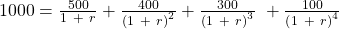r = 14.49%

A much faster method is to use the calculator:

 Key strokes Display [CF][2nd][CLR WORK] CF0 = 0 1000[+|-] [ENTER] CF0 = -1000 [↓] 500 [ENTER] C01 = 500 [↓] F01 = 1 [↓] 400 [ENTER] C02 = 400 [↓] F02 = 1 [↓] 300 [ENTER] C03 = 300 [↓] F03 = 1 [↓] 100 [ENTER] C04 = 100 [↓] F04 = 1 [IRR][CPT] 14.49

Project B: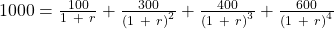r = 11.79%

Microsoft Excel functions can also be used to solve for the IRR. The two functions available are:

• IRR or =IRR(values, guess)
• XIRR or =XIRR(values, dates, guess)

where “values” are the cash flows, “guess” is an optional user-specified guess that defaults to 10%, and “dates” are the dates of each cash flow.

Ranking conflicts between NPV and IRR

For single and independent projects with conventional cash flows, there is no conflict between NPV and IRR decision rules. However, for mutually exclusive projects the two criteria may give conflicting results. The reason for conflict is due to differences in cash flow patterns and differences in project scale.

For example, consider two projects one with an initial outlay of $1 million and another project with an initial outlay of$1 billion. It is possible that the smaller project has a higher IRR, but the increase in firm value (NPV) is small as compared to the increase in firm value (NPV) of the larger project.

In case of a conflict, we should always go with the NPV criterion because:

• The NPV is a direct measure of expected increase in value of the firm.
• The NPV assumes reinvestment of cash flows at the required rate of return (more realistic), whereas the IRR assumes reinvestment of cash flows at the IRR rate (less realistic).
• IRR is not useful for projects with non-conventional cash flows as such projects can have multiple IRRs , i.e., there are more than one discount rates that will produce an NPV equal to zero.

Comparison between NPV and IRR

 NPV IRR Advantages Advantages Direct measure of expected increase in value of the firm. Shows the return on each dollar invested. Theoretically the best method. Allows us to compare return with the required rate. Disadvantages Disadvantages Does not consider project size. Incorrectly assumes that cash flows are reinvested at IRR rate. The correct assumption is that intermediate cash flows are reinvested at the required rate. Might conflict with NPV analysis. Possibility of multiple IRRs.

** IFT Website Un-available on Monday 2-October-2023, 0700-1200 GMT **
This is default text for notification bar